I will use this post to show you how, creating an AXI IP, we can accelerate our applications on Petalinux, or in case we use a Zynq MPSOC, we also can accelerate an application running in the RPU.

As you sure know, any kind of processor can execute only one instruction per core each clock cycle, and most of then, uses more than one cycle to execute only one instruction. That is because when we want to execute an instruction, processor, first has to access memory to read the instruction, then, if the instruction use data, has to access memory to read data, execute the instruction over data, and finally write data into memory. For improve this timing, processors has 4 stage pipeline, that means processor has precharged the 4 stages for execute the instruction, and then is can be executed in one cycle. Other kind of processors has only a 2 stages pipeline, so they spend 2 clock cycles for execute 1 instruction. In case of Zynq and Zynq MPSOC, both has a 4 stages pipeline, so each instruction uses only one clock cycle. Notice that I’ve use the word instruction, and not operation, because even if the processor could execute one instruction every clock cycle, most mathematical operation use several instruction for been executed. That, for signal processing, is translated in a high amount of time spent in operations, and this time will be decisive to determine the maximum frequency that we can sample. But relax, there are no problem, on Zynq, the PL has arrive to save us.

An FPGA works completely different, there are not instruction, only clock cycles for sequential designs and propagation delays for combinational. Also, FPGA has 2 great advantages respect a processor, first is the possibility of use custom width in our data, that made us save time and space, and the second one, the processes inside FPGA can be parallelized, multiplying the compute power.

The example that I’ve design, will show you how we can accelerate some algorithms, executing them partially or entirely on FPGA, and the time saved that we can achieve. The algorithm that I will use as an example is an iterative square root finder. This algorithm is used for compute an integer square root of a value. The algorithm, performs an iterative loop multiplying each number by itself, and the result is compared with the input value. When the result number is greater than the input value, the square root result is corresponding with the value that we multiplied by itself less 1. Each iteration of the algorithm will perform a multiplication, and a comparison, so the duration of the algorithm will depend of the input number, since the square root of the input number will be the same that the multiply and compare operations. The time spent on the execution of this algorithm on APU or RPU, will be the time that processor spends in execute this instructions. On the other hand, the time that our acceleration will spend to execute the same algorithm, not only depends of the PL itself. In this case, the processor has to send input value through AXI to the PL, then PL will execute the algorithm, and then, data will return to the processor. This kind of acceleration is very useful when time spent in data interchange is significantly lower than the execution time, in other case, the acceleration can be transformed in deceleration. In the next figure I will show you what I talking about.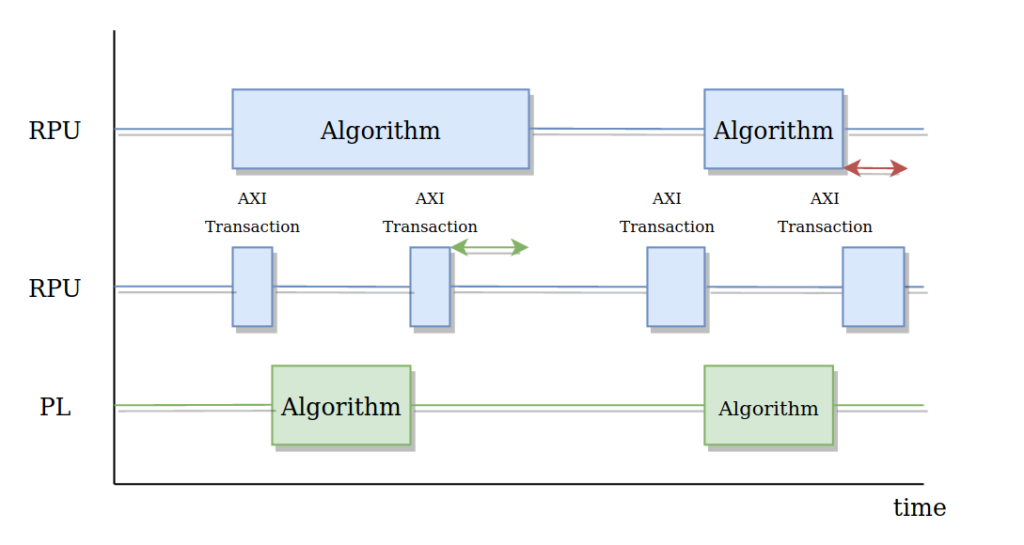In the example that I propose, as the duration of the algorithm depends of the input value, we can experiment with different amount of multiplication cycles.

For this example I will use Genesys ZU board, running the code in Zynq MPSOC. C code will be executed this time in the RPU. This Real Time Processor runs up to 600MHz. Algorithm code for the RPU is the next.

while(root_finder < root_to_find){
root_finder = square_root_result*square_root_result;
square_root_result +=1;
}


As I said, we are going to multiplying the index by itself until the result will be greater than the value that we want to find the root. In Verilog, code is similar, but there are an input bit from AXI that starts the algorithm, and one bit from the IP to PS to inform that the result is available in data address. You can find the code here.

First test I do is measure the time used in transfer data and manage the GPIO, for that, I’ve set the value that we want to know the root square to 0, so the PL only will perform 1 operation and as 0*0 is not less that 0, PL will set the end bit, and the PS will receive data. In the next figure we can see the state on the GPIO. The first pulse is the time that algorithm spends on execute itself. This time include the execution time and the transfers time. The second pulse is the time that algorithms spends is be executed on the PS. In this case, the transfer time is higher than the execution time, so this time, we can talk of deceleration.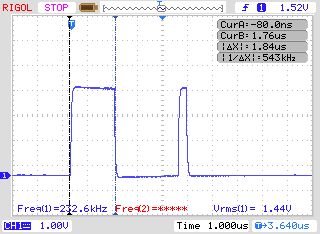I going to analyze the last capture. In that case, almost the entire time spend for our “accelerator” is corresponding to the transfer time. Obviously, this time also include the delay introduced by the GPIO, but that time is the same in the PS execution, so we will ignore it. On the other hand, the PS will spent arround 500ns to execute 1 operation. Making some spoiler, we can affirm that the PS time will be increased linearly with the number of operation, on the contrary, the spent time by the accelerator that we can see in this capture is a kind of offset, so the increase that we can expect is not linear. We will test again the accelerator but this time we want to find the root of 300, that is 17,32. The algorithm, in this case, will have to perform 18 multiplications.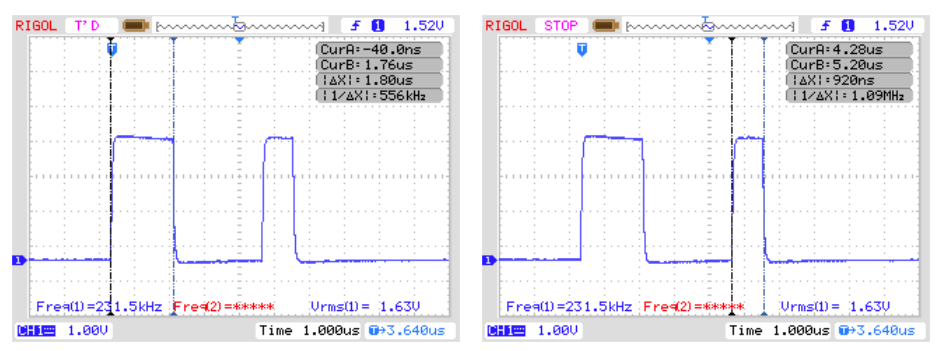We can see that the accelerator time is almost the same (even lower due to the resolution of the cursors), however, time spent by the PS is almost twice than before. I said before that the time spent in manage GPIO is too small for take it in count, but in last test, where time spent for the PS is so low, this time must be considered, and that’s the reason because PS time is not 18 times greater than before. In the next test, we want found the root of 5000. This time, the algorithm will perform 70 multiplications.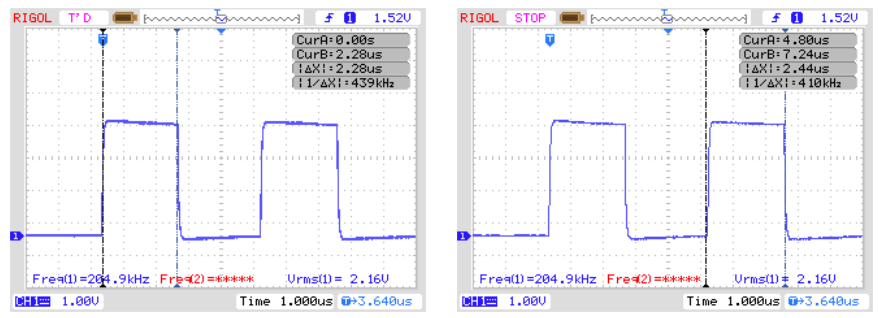This time, we can see that time spent by the PS is greater than time used by the PL. The small difference between 2 methods, means that this amount of operation is the limit in which our accelerators works as accelerator, and not as decelerator. In the next diagram, we can see this clearly. In this case we want to found the root of 10000.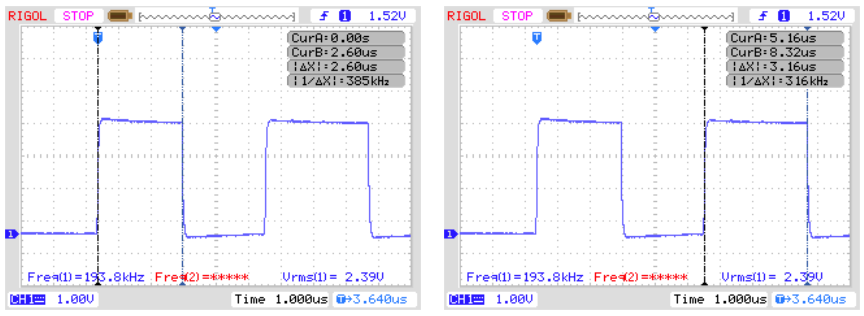This time, as you will guess, the PL accelerator is faster than the execution on the PS, and even 100 operations seems a high number, it is false, because 100 operation is the equivalent to a 32 order fir filter executing only 3 samples. If we want to filter a 128 samples signal, acceleration will make us save a lot of time, and that is traduced in a increase of sampling frequency.

I know that this is a very simple example of acceleration but it show the power of the acceleration using FPGA. This is a powerful tool in signal processing because if we want to execute a filter, or some transformation at the edge, we sure need accelerate the mathematical operations. Also, acceleration is often used for video processing because the algorithm has to process each bit separately, and this task will be so fast if we can parallelize this process with a significant amount of accelerators. Thanks for read!